## Cross Reference Down

#### Nagasaka, K, Kanemitsu, S and Shiue, JS (1990). Benford’s law: The logarithmic law of first digit. In: Győry, K, Halász, G. (eds.) Number theory. Vol. I. Elementary and analytic, Proc. Conf., Budapest/Hung. 1987, Colloq. Math. Soc. János Bolyai 51, pp. 361-391 .

This work cites the following items of the Benford Online Bibliography:

 Cohen, DIA and Katz, TM (1984). Prime Numbers and the First Digit Phenomenon. Journal of Number Theory 18(3), pp. 261-268. ISSN/ISBN:0022-314X. DOI:10.1016/0022-314X(84)90061-1.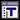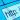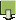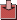Diaconis, P (1977). The Distribution of Leading Digits and Uniform Distribution Mod 1. Annals of Probability 5(1), pp. 72-81. ISSN/ISBN:0091-1798.Duncan, RL (1967). An application of uniform distributions to the Fibonacci numbers. Fibonacci Quarterly 5, pp. 137-140.Duncan, RL (1969). Note on the initial digit problem. Fibonacci Quarterly 7(5), pp. 474-475.Flehinger, BJ (1966). On the Probability that a Random Integer has Initial Digit A. American Mathematical Monthly 73(10), pp. 1056-1061. ISSN/ISBN:0002-9890. DOI:10.2307/2314636.Jager, H and Liardet, P (1988). Distribution arithmétiques des dénominateurs de convergents de fractions continues. Nederl. Akad. Wetensch. Indag. Math. 50(2), pp. 181-197. DOI:10.1016/S1385-7258(88)80026-X. FREKanemitsu, S, Nagasaka, K, Rauzy, G and Shiue, JS (1988). On Benford’s law: the first digit problem. Lecture Notes in Mathematics 1299, pp. 158-169 (eds. Watanabe, S, and Prokhorov, YV). ISSN/ISBN:978-3-540-18814-8. DOI:10.1007/BFb0078471.Katz, TM and Cohen, DIA (1986). The first digit property for exponential sequences is independent of the underlying distribution. Fibonacci Quarterly 24(1), pp. 2-7.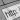Knopfmacher, J (1981). Initial digits in number theory. Fibonacci Quarterly 19(2), pp. 121-126.Kuipers, L (1969). Remark on a paper by R.L. Duncan concerning the uniform distribution mod 1 of the sequence of the logarithms of the Fibonacci numbers. Fibonacci Quarterly 7, pp. 465-466, 473.Kuipers, L and Niederreiter, H (1974). Uniform Distribution of Sequences. J. Wiley; newer edition - 2006 from Dover. ISSN/ISBN:0486450198.Kuipers, L and Shiue, JS (1973). Remark on a paper by Duncan and Brown on the sequence of logarithms of certain recursive sequences. Fibonacci Quarterly 11(3), pp. 292-294.Nagasaka, K (1984). On Benford's Law. Annals of the Institute of Statistical Mathematics 36(2), pp. 337-352. ISSN/ISBN:0020-3157. DOI:10.1007/BF02481974.Nagasaka, K and Shiue, JS (1987). Benford's law for linear recurrence sequences. Tsukuba Journal of Mathematics 11(2), pp. 341-351.Schatte, P (1988). On the uniform distribution of certain sequences and Benford’s law. Math. Nachr. 136, 271-273. DOI:10.1002/mana.19881360119.Schatte, P (1990). On Benford’s law for continued fractions. Math. Nachr. 148, 137-144. DOI:10.1002/mana.3211480108.Schatte, P and Nagasaka, K (1991). A note on Benford’s law for second order linear recurrences with periodical coefficients. Z. Anal. Anwend. 10(2), pp. 251-254.